> 文档中心 > 独孤九剑第四式-K近邻模型(KNN)

# 独孤九剑第四式-K近邻模型(KNN)

💐文章适合于所有的相关人士进行学习💐
🍀各位看官看完了之后不要立刻转身呀🍀
🌿期待三连关注小小博主加收藏🌿
🍃小小博主回关快 会给你意想不到的惊喜呀🍃

### 文章目录

• 🐢前言
• 🐢KNN理论讲解
• 🐧模型思想
• 🐧确定K值
• 🐔欧氏距离
• 🐔曼哈顿距离
• 🐔余弦相似度
• 🐢实战演练
• 🐧数据展示
• 🐧读入
• 🐧拆分为训练集和测试集
• 🐧确定K值
• 🐧构造混淆矩阵
• 🐧热力图## 🐢前言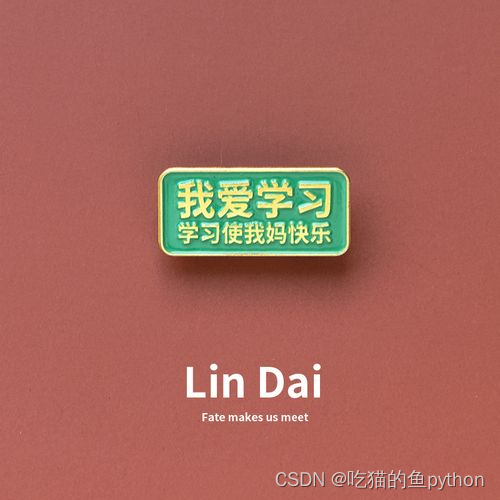## 🐢KNN理论讲解

#### 🐧模型思想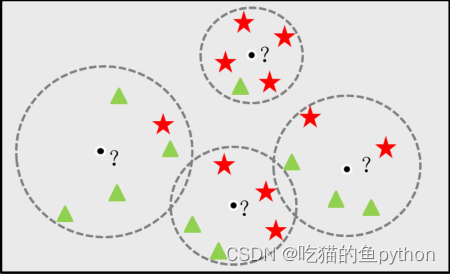#### 🐧确定K值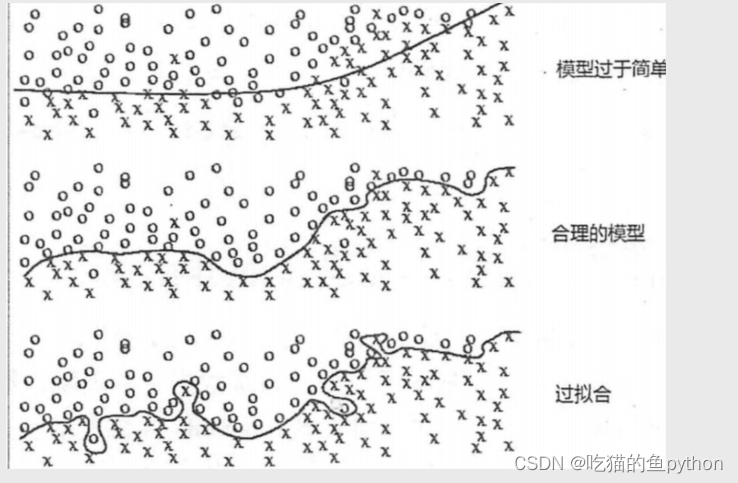设置k近邻样本的投票权重，使用KNN算法进行分类或预测时设置的k值比较大，担心模型发生欠拟合的现象，一个简单有效的处理办法就是设置近邻样本的投票权重，如果已知样本距离未知样本比较远，则对应的权重就设置得低一些，否则权重就高一些，通常可以将权重设置为距离的倒数。

 我们之前也学过10重交叉验证法这种方式来去解决选择参数这个问题，那么这个K值得选择我们也可以进行这样选择，方式就是我们对于每一个K值，都进行多重交叉验证。每一重都选出计算出其平均误差，然后进行比较就得出了最后得K值。

``import numpy as npfrom sklearn import neighborsimport matplotlib.pyplot as plt# 设置K得数值，定义为int类型也就是整形K = np.arange(1,np.ceil(np.log2(Knowledge.shape))).astype(int)# 存储平均准确率accuracy = []for k in K:    # 使用交叉验证的方法，比对每一个k值下KNN模型的预测准确率    cv_result = model_selection.cross_val_score(neighbors.KNeighborsClassifier(n_neighbors = k, weights = 'distance'),X_train, y_train, cv = 10, scoring='accuracy')    accuracy.append(cv_result.mean())# 从k个平均准确率中挑选出最大值所对应的下标    arg_max = np.array(accuracy).argmax()# 中文和负号的正常显示plt.rcParams['font.sans-serif'] = ['Microsoft YaHei']plt.rcParams['axes.unicode_minus'] = False# 绘制不同K值与平均预测准确率之间的折线图plt.plot(K, accuracy)# 添加点图plt.scatter(K, accuracy)# 添加文字说明plt.text(K[arg_max], accuracy[arg_max], '最佳k值为%s' %int(K[arg_max]))# 显示图形plt.show()``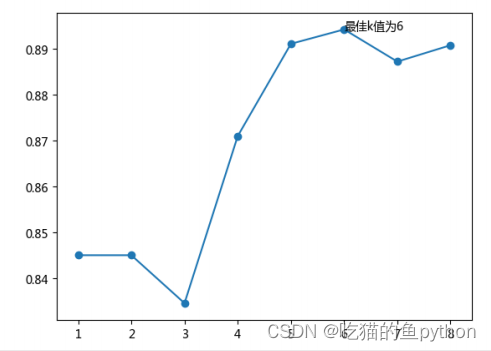##### 🐔欧氏距离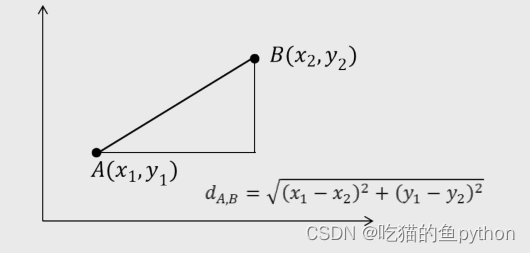##### 🐔曼哈顿距离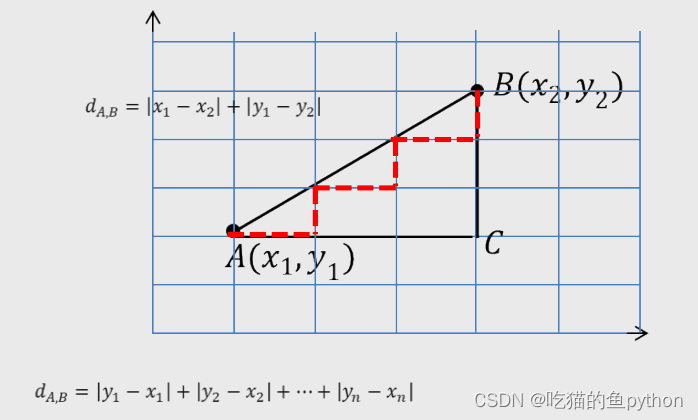##### 🐔余弦相似度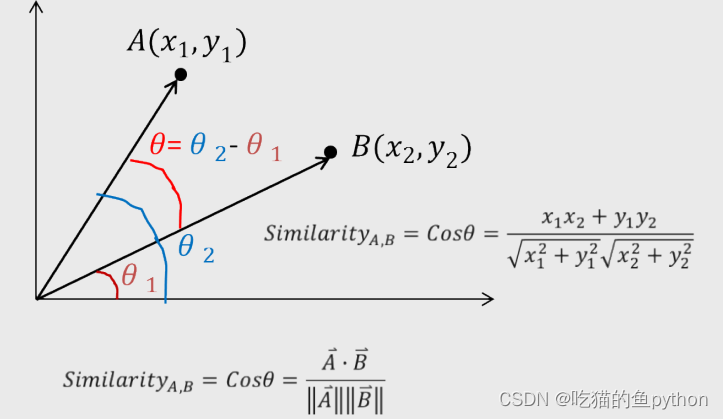## 🐢实战演练

#### 🐧数据展示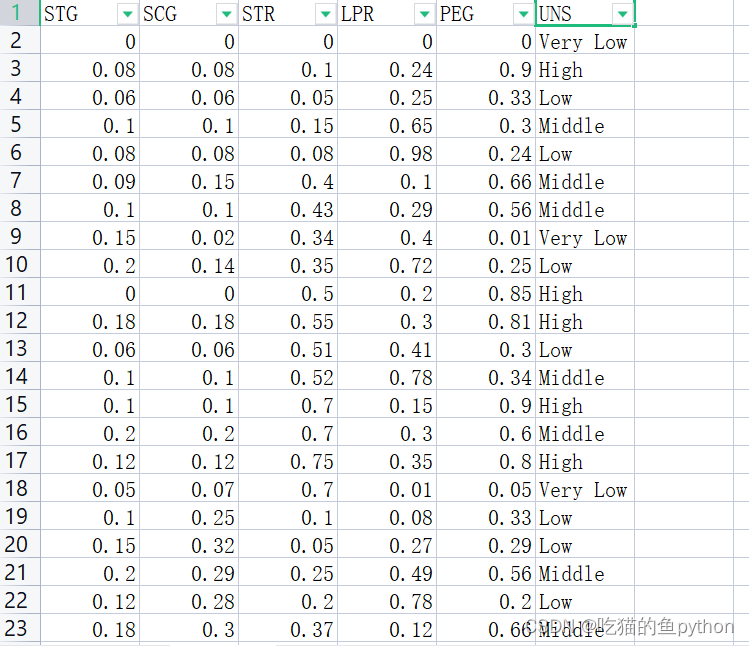#### 🐧读入

``import pandas as pdKnowledge = pd.read_excel(r'Knowledge.xlsx')Knowledge.head()``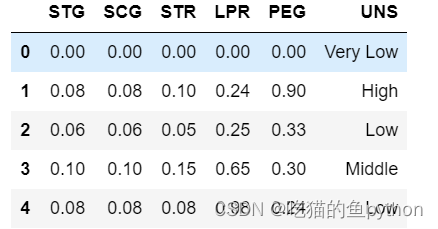#### 🐧拆分为训练集和测试集

``from sklearn import model_selection# 将数据集拆分为训练集和测试集predictors = Knowledge.columns[:-1]X_train, X_test, y_train, y_test = model_selection.train_test_split(Knowledge[predictors], Knowledge.UNS,      test_size = 0.25, random_state = 1234)``

#### 🐧确定K值

``import numpy as npfrom sklearn import neighborsimport matplotlib.pyplot as plt# 设置K得数值，定义为int类型也就是整形K = np.arange(1,np.ceil(np.log2(Knowledge.shape))).astype(int)# 存储平均准确率accuracy = []for k in K:    # 使用交叉验证的方法，比对每一个k值下KNN模型的预测准确率    cv_result = model_selection.cross_val_score(neighbors.KNeighborsClassifier(n_neighbors = k, weights = 'distance'),X_train, y_train, cv = 10, scoring='accuracy')    accuracy.append(cv_result.mean())# 从k个平均准确率中挑选出最大值所对应的下标    arg_max = np.array(accuracy).argmax()# 中文和负号的正常显示plt.rcParams['font.sans-serif'] = ['Microsoft YaHei']plt.rcParams['axes.unicode_minus'] = False# 绘制不同K值与平均预测准确率之间的折线图plt.plot(K, accuracy)# 添加点图plt.scatter(K, accuracy)# 添加文字说明plt.text(K[arg_max], accuracy[arg_max], '最佳k值为%s' %int(K[arg_max]))# 显示图形plt.show()``

#### 🐧构造混淆矩阵

``from sklearn import metrics# 重新构建模型，并将最佳的近邻个数设置为6knn_class = neighbors.KNeighborsClassifier(n_neighbors = 6, weights = 'distance')#权重设置为距离knn_class.fit(X_train, y_train)predict = knn_class.predict(X_test)cm = pd.crosstab(predict,y_test)cm#构造混淆矩阵``

#### 🐧热力图

``import seaborn as sns# 将混淆矩阵构造成数据框，并加上字段名和行名称，用于行或列的含义说明cm = pd.DataFrame(cm)sns.heatmap(cm, annot = True,cmap = 'GnBu')plt.xlabel(' Real Lable')plt.ylabel(' Predict Lable')plt.show()``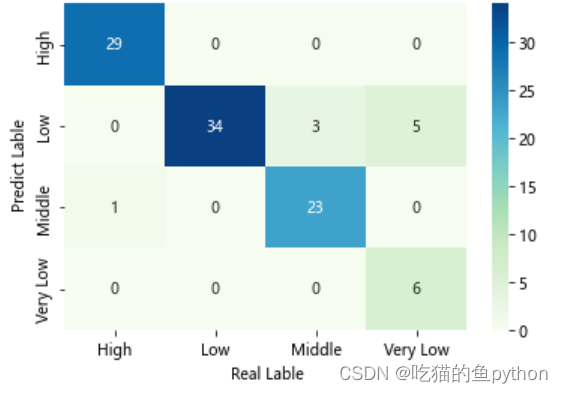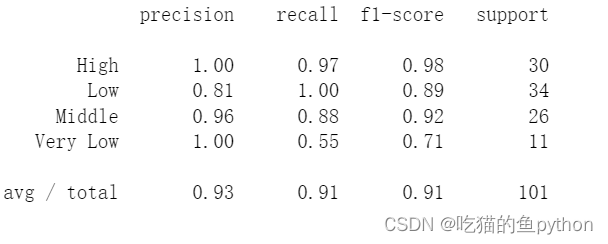💐文章适合于所有的相关人士进行学习💐
🍀各位看官看完了之后不要立刻转身呀🍀
🌿期待三连关注小小博主加收藏🌿
🍃小小博主回关快 会给你意想不到的惊喜呀🍃